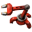## Production Challenge

Place to share custom user maps, scenarios and campaigns.
henke37
Long Handed InserterPosts: 89
Joined: Mon Jul 18, 2016 5:43 pm
Contact:

### Production Challenge

Since noone else seems to be interested in making the topic, I will.

For those who missed it, the production challenge is an official multiplayer scenario where teams compete to be the first to reach an arbitrary production goal. The game is played over several rounds, usually five or ten. Each round has its own goal and conditions. Completing each round awards points to the team, with decreasing point gains as other teams complete the goal before you.

Each team has their own square of the map. Each square is identical and they are all reset before each round. There is an iron ore field in the south west, a copper field in the north east, a coal field in the north west and a stone field in the south east. At the top and bottom of the map is the drop off chests, put the produced items in them. Next to the chests is a power pole and an accumulator. The accumulator has a practically infinite charge, so power is free. The map has no oil and no biters. And it's always day.

The rounds differ in the production goal as well as the initial items each player gets. Usually round goals are about producing one to three types of items. Each item has its own base count, but the number of item categories for the round and randomness modulate it.

Once in eight, the round goal is not to produce items, but to research one to three research items.

Each player gets one of three starting inventories, selected globaly at random at the round start. There is also a 30 % chance of getting a power armor with construction bots.

Code: Select all

``````global.disabled_items =
{
"submachine-gun",
"pistol",
"shotgun",
"combat-shotgun",
"rocket-launcher",
"land-mine",
"poison-capsule",
"slowdown-capsule",
"flame-thrower",
"distractor_capsule",
"destroyer_capsule",
"rocket",
"flame-thrower-ammo",
"laser-turret",
"night-vision-equipment",
"solar-panel-equipment",
"energy-shield-equipment",
"battery-equipment"
}

global.item_list =
{
{name = "copper-cable", count = 1000},
{name = "iron-stick", count = 400},
{name = "iron-plate", count = 300},
{name = "copper-plate", count = 300},
{name = "pipe", count = 300},
{name = "transport-belt", count = 250},
{name = "iron-gear-wheel", count = 200},
{name = "electronic-circuit", count = 150},
{name = "stone-furnace", count = 100},
{name = "stone-brick", count = 100},
{name = "medium-electric-pole", count = 100},
{name = "red-wire", count = 100},
{name = "green-wire", count = 100},
{name = "boiler", count = 100},
{name = "repair-pack", count = 100},
{name = "firearm-magazine", count = 100},
{name = "shotgun-shell", count = 100},
{name = "burner-mining-drill", count = 100},
{name = "concrete", count = 300},
{name = "assembling-machine-1", count = 50},
{name = "science-pack-1", count = 50},
{name = "splitter", count = 50},
{name = "iron-axe", count = 50},
{name = "steel-plate", count = 50},
{name = "rail", count = 50},
{name = "fast-inserter", count = 50},
{name = "long-handed-inserter", count = 50},
{name = "empty-barrel", count = 50},
{name = "rail-signal", count = 50},
{name = "train-stop", count = 50},
{name = "arithmetic-combinator", count = 50},
{name = "steel-chest", count = 50},
{name = "small-lamp", count = 50},
{name = "lab", count = 50},
{name = "train-stop", count = 50},
{name = "fast-transport-belt", count = 50},
{name = "landfill", count = 50},
{name = "stone-wall", count = 50},
{name = "gate", count = 50},
{name = "radar", count = 50},
{name = "light-armor", count = 50},
{name = "piercing-rounds-magazine", count = 50},
{name = "assembling-machine-2", count = 25},
{name = "steel-axe", count = 25},
{name = "defender-capsule", count = 25},
{name = "electric-mining-drill", count = 25},
{name = "steam-engine", count = 25},
{name = "science-pack-2", count = 25},
{name = "steel-furnace", count = 25},
{name = "engine-unit", count = 25},
{name = "big-electric-pole", count = 25},
{name = "gun-turret", count = 25},
{name = "solar-panel", count = 25},
{name = "chemical-plant", count = 25},
{name = "filter-inserter", count = 25},
{name = "fast-splitter", count = 25},
{name = "fast-underground-belt", count = 25},
{name = "piercing-shotgun-shell", count = 25}
}
global.starting_inventories =
{
["small"] =
{
{name = "iron-plate", count = 20},
{name = "copper-plate", count = 10},
{name = "iron-axe", count=1},
{name = "burner-mining-drill", count=5},
{name = "stone-furnace", count=5},
{name = "burner-inserter", count=15},
{name = "transport-belt", count=40},
},
["medium"] =
{
{name = "iron-plate", count = 50},
{name = "iron-gear-wheel", count = 50},
{name = "copper-plate", count = 50},
{name = "electronic-circuit", count = 50},
{name = "transport-belt", count = 150},
{name = "inserter", count = 50},
{name = "burner-inserter", count=50},
{name = "transport-belt", count=200},
{name = "small-electric-pole", count=20},
{name = "burner-mining-drill", count=20},
{name = "electric-mining-drill", count=8},
{name = "stone-furnace", count=20},
{name = "steel-furnace", count=8},
{name = "assembling-machine-1", count=20},
{name = "iron-axe", count=3}
},
["large"] =
{
{name = "iron-plate", count = 50},
{name = "copper-plate", count = 50},
{name = "steel-plate", count = 50},
{name = "transport-belt", count = 250},
{name = "inserter", count = 50},
{name = "burner-inserter", count=50},
{name = "small-electric-pole", count=50},
{name = "burner-mining-drill", count=50},
{name = "electric-mining-drill", count=20},
{name = "stone-furnace", count=35},
{name = "steel-furnace", count=20},
{name = "electric-furnace", count=8},
{name = "steel-axe", count=3},
{name = "assembling-machine-1", count = 50},
{name = "assembling-machine-2", count = 20},
{name = "iron-gear-wheel", count = 50},
{name = "electronic-circuit", count = 50},
{name = "fast-inserter", count = 30},
{name = "medium-electric-pole", count = 30},
}
}
``````